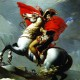# Scope too narrow?

Not sure if I should be using the event or the i variable because either way it either boldens everything or nothing.

app.js
```const laws = document.getElementsByTagName('li');
const indexText = document.getElementById('boldIndex');
const button = document.getElementById('embolden');

const index = parseInt(indexText.value, 10);

for (let i = 0; i < laws.length; i += 1) {
let law = laws[i];

// replace 'false' with a correct test condition on the line below
if (event.textInput === 0,1,2) {

law.style.fontWeight = 'bold';
} else {
law.style.fontWeight = 'normal';
}
}
});
```
index.html
```<!DOCTYPE html>
<html>
<title>Newton's Laws</title>
<body>
<h1>Newton's Laws of Motion</h1>
<ul>
<li>An object in motion tends to stay in motion, unless acted on by an outside force.</li>
<li>Acceleration is dependent on the forces acting upon an object and the mass of the object.</li>
<li>For every action, there is an equal and opposite reaction.</li>
</ul>
<input type="text" id="boldIndex">
<button id="embolden">Embolden</button>
<script src="app.js"></script>
</body>
</html>
```Ian,

1) The event is designated 'e' on line 5, not 'event'. (But this isn't needed for the answer)

2) The button was clicked that created the even so e.textInput would be pulling information from the button not the input box.

3) The input box was put into a variable already on line 2

```const indexText = document.getElementById('boldIndex');
```

4) You are in a loop, so you can use the i variable instead of 0,1,2 on line 12

5) So check to see if indexText is equal to the index of the loop.

``` if (indexText === i) {